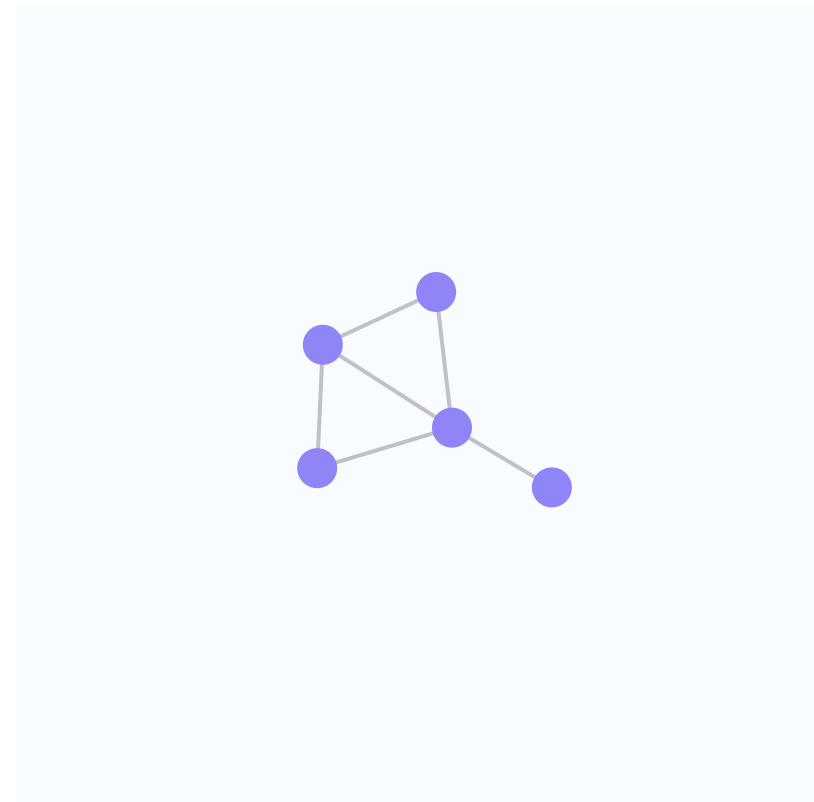# Automatic layout for VX Network with D3.js

I’ve lately used vx visualization components to implement different data visualizations. It provides small pieces you combine and customize to build your own custom visualizations. I’ve found it quite comprehensible and deeply customizable.

vx includes a network graph component for showing graph data which I needed for a project. Unfortunately it doesn’t automatically calculate the positions for the graph nodes, so I decided to use the D3.js force simulation to calculate the X and Y coordinates for the nodes.React component’s `componentDidMount` callback sets up d3 `forceSimulation` which takes care of setting the `x` and `y` properties for the passed nodes.

The full code for a simple network graph with automatic layout is below. You can also view how it looks and play around with it in CodeSandbox.

``````import React from "react";
import ReactDOM from "react-dom";

import { Graph } from "@vx/network";
import {
forceCenter,
forceManyBody,
forceSimulation
} from "d3-force";

// The node rendered by the graph
class NetworkNode extends React.Component {
render() {
return <circle r={10} fill={"#9280FF"} />;
}
}

class Network extends React.Component {
constructor(props) {
super(props);

const nodes = props.network.nodes;

this.state = {
data: {
nodes,
}
};
}

// Update force if the width or height of the graph changes
componentDidUpdate(newProps) {
if (
newProps.width !== this.props.width ||
newProps.height !== this.props.height
) {
this.force = this.force
.force("center", forceCenter(
newProps.width / 2,
newProps.height / 2
))
.restart();
}
}

// Setup D3 force
componentDidMount() {
this.force = forceSimulation(this.state.data.nodes)
.force(
.id(function(d) {
return d.id;
})
)
.force("charge", forceManyBody().strength(-500))
.force(
"center",
forceCenter(this.props.width / 2, this.props.height / 2)
);

// Force-update the component on each force tick
this.force.on("tick", () => this.forceUpdate());
}

render() {
if (!this.force) {
return null;
}

return (
<div style={{ width: "100%", height: "100%" }}>
<svg width={this.props.width} height={this.props.height}>
<rect
width={this.props.width}
height={this.props.height}
fill="#f9fcff"
/>
<Graph graph={this.state.data} nodeComponent={NetworkNode} />
</svg>
</div>
);
}
}

function App() {
const nodes = [{ id: 1 }, { id: 2 }, { id: 3 }, { id: 4 }, { id: 5 }];
{ source: 1, target: 2 },
{ source: 1, target: 3 },
{ source: 1, target: 4 },
{ source: 2, target: 4 },
{ source: 3, target: 4 },
{ source: 4, target: 5 }
];
return (
<div className="App">
<Network
width={400}
height={400}
network={{
nodes: nodes,